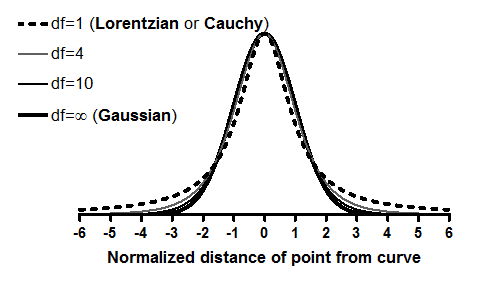## Please enable JavaScript to view this site.

 Robust nonlinear regression

## The need for robust regression

Nonlinear regression, like linear regression, assumes that the scatter of data around the ideal curve follows a Gaussian or normal distribution. This assumption leads to the familiar goal of regression: to minimize the sum of the squares of the vertical or Y-value distances between the points and the curve. This standard method for performing nonlinear (or linear regression) is called least-squares.

Experimental mistakes can lead to erroneous values whose values are way too high or too low– outliers. Even a single outlier can dominate the sum-of-the-squares calculation, and lead to misleading results. One way to cope with this problem is to perform a robust fit using a method that is not very sensitive to violations of the Gaussian assumption. Another approach is to use automatic outlier elimination to identify and remove the outliers, and then run least-squares regression. Prism offers both choices. The outlier identification method actually first does a robust fit, so it has a baseline from which to decide when a point is too far from that baseline so becomes defined as an outlier. Then after removing those outliers, it  it performs a standard least-squares fit on the remaining points.

## How robust regression works

Based on a suggestion in Numerical Recipes (1), we based our robust fitting method on the assumption that variation around the curve follows a Lorentzian distribution, rather than a Gaussian distribution. Both distributions are part of a family of t distributions:The widest distribution in that figure, the t distribution for df=1, is also known as the Lorentzian distribution or Cauchy distribution. The Lorentzian distribution has wide tails, so outliers are fairly common and therefore have little impact on the fit.

We adapted the Marquardt nonlinear regression algorithm to accommodate the assumption of a Lorentzian (rather than Gaussian) distribution of residuals, and explain the details in reference 2.

## When does it make sense to choose robust nonlinear regression?

The main use of robust regression in Prism is as a 'baseline' from which to remove outliers. You may want to experiment with robust regression in order to better understand the outlier-removal method (which begins with robust regression). You may also find it useful if your only goal is to interpolate from a standard curve, and that standard curve has one or more outliers.

We recommend that you avoid robust regression (at least as implemented by Prism) for most uses, because it has these  drawbacks:

Robust regression cannot calculate standard errors or confidence intervals for the parameters.

Robust regression cannot plot confidence or prediction bands.

Robust regression cannot compute the degree to which parameters are intertwined. It cannot compute the covariance matrix or the dependency values.

Robust regression cannot compare the fits of two models or two data sets.

The inability to compare models does not matter in most contexts. But the inability to provide diagnostic information (SE, CI, Covariance matrix, Dependency, Confidence and prediction bands) seriously limits the usefulness of robust regression for most research.

## References

1.Press WH, Teukolsky SA, Vettering WT, Flannery BP: Numerical Recipes in C. the Art of Scientific Computing. New York, NY: Cambridge University Press; 1988.

2.Motulsky HM and Brown RE, Detecting outliers when fitting data with nonlinear regression – a new method based on robust nonlinear regression and the false discovery rate, BMC Bioinformatics 2006, 7:123. Download as pdf.